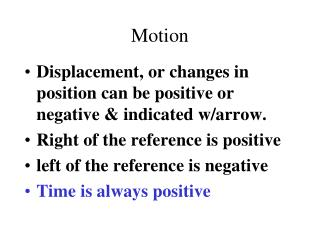DownloadDownload PresentationMotion

# Motion

Download Presentation## Motion

- - - - - - - - - - - - - - - - - - - - - - - - - - - E N D - - - - - - - - - - - - - - - - - - - - - - - - - - -
##### Presentation Transcript

1. Motion • Displacement, or changes in position can be positive or negative & indicated w/arrow. • Right of the reference is positive • left of the reference is negative • Time is always positive

2. Uniform Motion

3. Non-uniform motion

4. Motion w/ constant acceleration

5. Motion w/ constant acceleration

6. Motion w/ constant acceleration

7. Falling object in air

8. A bouncing ball

9. Motion in a straight line

10. Never connect the dots if the points do not form a smooth line, • draw the best smooth curve possible. • App. The same # above & below the line.

11. Interpolation- reading from the graph between data points • Extrapolation- reading the graph beyond the limits

12. Use + and – to indicate the position in relationship to the reference point. • We can use – points!

13. Sketch position-time graphs for these four motions:a. starting at a positive position with a positive velocityb. starting at a negative position with a smallerpositive velocityc. remaining at a negative positiond starting at a positive position with anegative velocity

14. . 1st 5 sec- increase next 5 s- runs at a a constant 8 m/s last 3 sec. - slows to a stop How would you describe the motion of the runner shown in the graph. The vertical value of any point is the instantaneous velocity at that time

15. Draw a position-time graph of a moving elevator. Use the first floor as reference point and up as positive. The elevator waits on the 1st floor for 30 s, rises to the third floor in 20s, where it stops for 30 s, before going to the basement, which it reaches in 40 s.

16. Instantaneous position • Position at the moment • Moving objects have a series of instantaneous Positions.

17. Time interval • t = t 2 - t 1 • The triangle (delta) stands for change & is used in many measurements

18. Independent- X • Y • Dependent

19. - Linear - straight line y = mx + b m & b are constants Dependent variable varies directly w/independent

20. If y varies inversely with x- Hyberbola xy= k Boyle’s law- When the Pressure of a gas is doubled, the volume will be reduced to 1/2 its original volume

21. Y varies directly w/square of x; • Parabola • y = kx2

22. Slope • The displacement is the vertical separation Rise • the time is the horizontal separation-Run • The ratio of the rise to the run • use the largest possible rise & run for more accuracy

23. Backing up- the acceleration is negative. • When it comes to a stop the final velocity will be less negative than the initial velocity. • The acceleration will be positive even though the car is moving slower.

24. Position- time graphs indicate velocity • velocity-time graphs indicate acceleration

25. Uniform or constant Acceleration • Does not change • velocity-time graph = straight line • initial velocity- when the clock reading is zero

26. Position-Time graph • Shows how position depends on clock reading or time • time is the independent variable • position is dependent variable • straight line used (best)

27. Acceleration- zero • Motion at constant speed

28. Motion at constant speed • Acceleration- zero

29. A position-time graph for uniformly accelerating motionparabola- varies directly w/square

30. Instantaneous Velocity • May use a position-time graph to determine • the slope at different times. • Is the tangent to a curve on a position-time graph

31. Velocity-Time Graphs • Describes motion with either constant or changing velocity • Model the motion with your hand • the displacement between 2 times is the area under the curve of a velocity-time graph

32. Velocity-time graphfor an airplane moving w/a constant velocity of 260 m/sVelocity is constant • The position-time graph

33. Constant velocity • The time interval is the same at all time intervals • called uniform velocity • the ratio of d/ t is constant • uniform velocity= • v = d t

34. Constant Velocity

35. Uniform Velocity

36. average velocity • The slope is the average velocity • V= d/t • slope = rise = d run  t

37. car Shifting w/four gears

38. Slope (acceleration) is greatest just as the car begins accelerating from rest. • The acceleration is smallest when the velocity is largest.

39. Constant acceleration • The straight line “curve” on a velocity-time graph indicates that the change in velocity is the same in each time interval • The slope (acceleration) is constant • The airplane acceleration = 20 m/s/s/. • Velocity inc. 20 m/s for every second

40. Constant Acceleration

41. Uniform Acceleration

42. Uniformly accelerated motion- airplane

43. The slope at any point on a position-time graph = the velocity of the object

44. The slope of a position-time graph is the velocity of the object • W/constant velocity/constant slope -straight linew/acceleration-parabola

45. The slope of a position-time graphw/acceleration -parabola • to find the slope of the curve at a given point draw a line tangent to the curve at that point • the slope of the tangent line is the instantaneous velocity